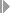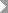Show TOC

###TRM, CRA, Calculation Method for Calculation Base of the NG

In Limit Management of the Credit Risk Analyzer, you use settings to specify which key figures flow into the calculation base for determining the attributable amounts of a netting group. For this, you can specify in Customizing under Define Determination Procedures (by choosing the Attributable Amount Calculation Method of Netting Group) whether all key figures - calculated for the individual transactions of the netting group - are considered in the calculation base of the netting group, or whether only positive or negative key figures are considered.

The calculation base of the netting group is relevant for calculating the attributable amount for the netting group.

#### Technical Details

Technical Name of the Product Feature TRM_CRA_NG_AA_METHOD New Valid for all countries EA-FINSERV 605 EA-FINSERV 606 EA-FINSERV 616 EA-FINSERV 617 FIN-FSCM-TRM-CR SAP enhancement package 5 (SP15) for SAP ERP 6.0 SAP enhancement package 6 (SP14) for SAP ERP 6.0 SAP enhancement package 6 for SAP ERP 6.0, version for SAP HANA, Support Package 09 SAP enhancement package 7 (SP07) for SAP ERP 6.0 TRM, New Instruments, Accounting Enhancements, Reporting (FIN_TRM_LR_FI_AN_3)

The system calculates the following totals for the netting group:

• Total NPV (Netting Transactions)

This is the total of all calculation bases calculated (in accordance with the settings of the determination procedure) for the individual transactions of the netting group.

• Total NPV (Netting Transactions with Positive NPV)

This is the total of all positive calculation bases calculated (in accordance with the settings of the determination procedure) for the individual transactions of the netting group.

• Total NPV (Netting Transactions with Negative NPV)

This is the total of all negative calculation bases calculated (in accordance with the settings of the determination procedure) for the individual transactions of the netting group.

You can use the calculation method in Customizing for the Credit Risk Analyzer to specify for the determination procedure which of these values is used for the calculation base of the netting group:

• 0 Positive and Negative Key Figures

All key figures are relevant for the calculation base of the netting group [-> calculation base of the netting group = Total NPV (Netting Transactions) + add-on netting group]

• 1 Only Positive Key Figures

In this case, only the positive key figures are relevant for the calculation base of the netting group [-> calculation base of the netting group = Total NPV (Netting Transactions with Positive NPV) + add-on netting group]

• 2 Only Negative Key Figures

In this case, only the negative key figures are relevant for the calculation base of the netting group [-> calculation base of the netting group = Total NPV (Netting Transactions with Negative NPV) + add-on netting group]

Using the calculation base BBAS of the netting group, the system calculates the attributable amount of the netting group.Note

• Now you can also use the user exit (function module EXIT_SAPLKLEX_001) to transfer attributable amounts for netting groups.

• The key figures Total NPV (Netting Transactions), Total NPV (Netting Transactions with Positive NPV), and Total NPV (Netting Transactions with Negative NPV) are also transferred to the user exit for calculation of the attributable amount, and you can decide within the user exit (function module EXIT_SAPLKLEX_001) which of the key figures flow into the calculation base.

End of the note.

#### Effects on Existing Data

The new setting affects how the attributable amounts of a netting group are calculated using the following functions:

#### Effects on Customizing

In Customizing for the Credit Risk Analyzer underBasic SettingsDefinitionsDefine Determination Procedures, there is the new field Attributable Amount Calculation Method (Attr.Amnt.Calc.Meth) in the Netting area.